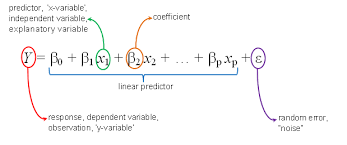# Beginning with ML 2.0: Multivariate Linear Regression

July 16, 2019 5164In my first blog, I covered the basics of Linear Regression with an example of online shopping brands trying to smartly invest in advertisements on social media. If you haven’t read that yet, here’s a link.

Now, let’s complicate our situation. Suppose the same companies want to calculate their investment to sales ratio as above, but with more social media platforms. Our dataset now looks like this.

 Social Media Instagram Facebook Twitter Company Investment Sales Investment Sales Investment Sales Myntra 50000 52500 30000 28500 25000 25100 Jabong 48000 49500 25000 24300 15000 14800 LimeRoad 51200 51000 28000 27500 18000 18000

This is where Multivariate Regression comes in. While Linear Regression has only one input feature, multivariate linear regression uses multiple features. Here, the algorithm is still trying to learn the best fit for investment-sales prediction but is now doing so for multiple social media platforms. While dealing with one input, our plot was a single line (unidimensional). Now, our plot will result in a plane (multidimensional). And thus, the equation of multivariate regression can be represented as follows:The introduction of more inputs into our model will naturally result in it becoming more accurate in general. However, it will also give rise to a bunch of new terms. On that note, let’s talk about a new term- ‘Feature Scaling’. While Feature Scaling doesn’t really apply to the situation we are considering, it is a key process in most machine learning applications. So, what is Feature Scaling? Consider another example. Suppose that you are looking to sell your mobile online, so that you can use that money to buy a newer one. Obviously, you’ll want to sell it at the maximum price possible. One way of doing this, is to look at the previous sales of second-hand mobiles online. Now, the most common parameters that are most likely to affect these sales would be the mobile brand, the price, the number of years it has been used, the storage capacity and the camera quality. While the price will be large number, the number of years will mostly be a single digit and the storage capacity measured in gigabytes will be two or three digits. Basically, each important feature has a different range. Thus, directly using these numbers for your Multivariate Linear Regression algorithm will make the model highly inaccurate. Feature scaling is hence used to normalise the range of independent features of data. It is also called Normalisation.

In Multivariate Linear Regression, it is important to perform feature scaling (wherever required) before computing the cost function. As seen in my last blog, the cost function is a mathematical expression which gives us the difference between the expected and obtained output. For multivariate Linear Regression, the cost function remains the same as that of Univariate Linear Regression.We already know that our algorithm will yield accurate results only if we perform normalization first. The question is, why? Because gradient descent works better with normalisation, reaching the global minimum faster. Recap: Gradient descent is an optimization algorithm that minimizes the cost function. The equations for gradient descent also remain the same as linear regression.Also like in linear regression, the gradient descent depends upon the learning rate, alpha. Consider the image below:Thus, the value of alpha should neither be too big nor too small. If the learning rate is too small, convergence may be too slow. However, if it is too large, divergence may occur, which will throw your algorithm completely off track.

Another noteworthy basic concept of multivariate linear regression is feature selection. The thing is, our algorithm will not do well if it is given too many features, nor will it do well if it is given too few features. Too many features will cause overfitting, ie the algorithm will be too focused on making the right prediction for each example in the dataset. As a result, it will probably give an incorrect output when given new data. On the other hand, if the algorithm is provided with too few features, it will cause under-fitting. Simply put, it will mean that our algorithm requires more data. Thus, it is of utmost importance to provide the right amount of data while training our algorithm.

That’s it for Multivariate Linear Regression! Check out my next post for Logistic Regression. Thanks for reading!

Liked the post?
I'm an engineer and writer, passionate about Artificial Intelligence. Looking to change the world with a combination of innovation and kindness.
0 COMMENT